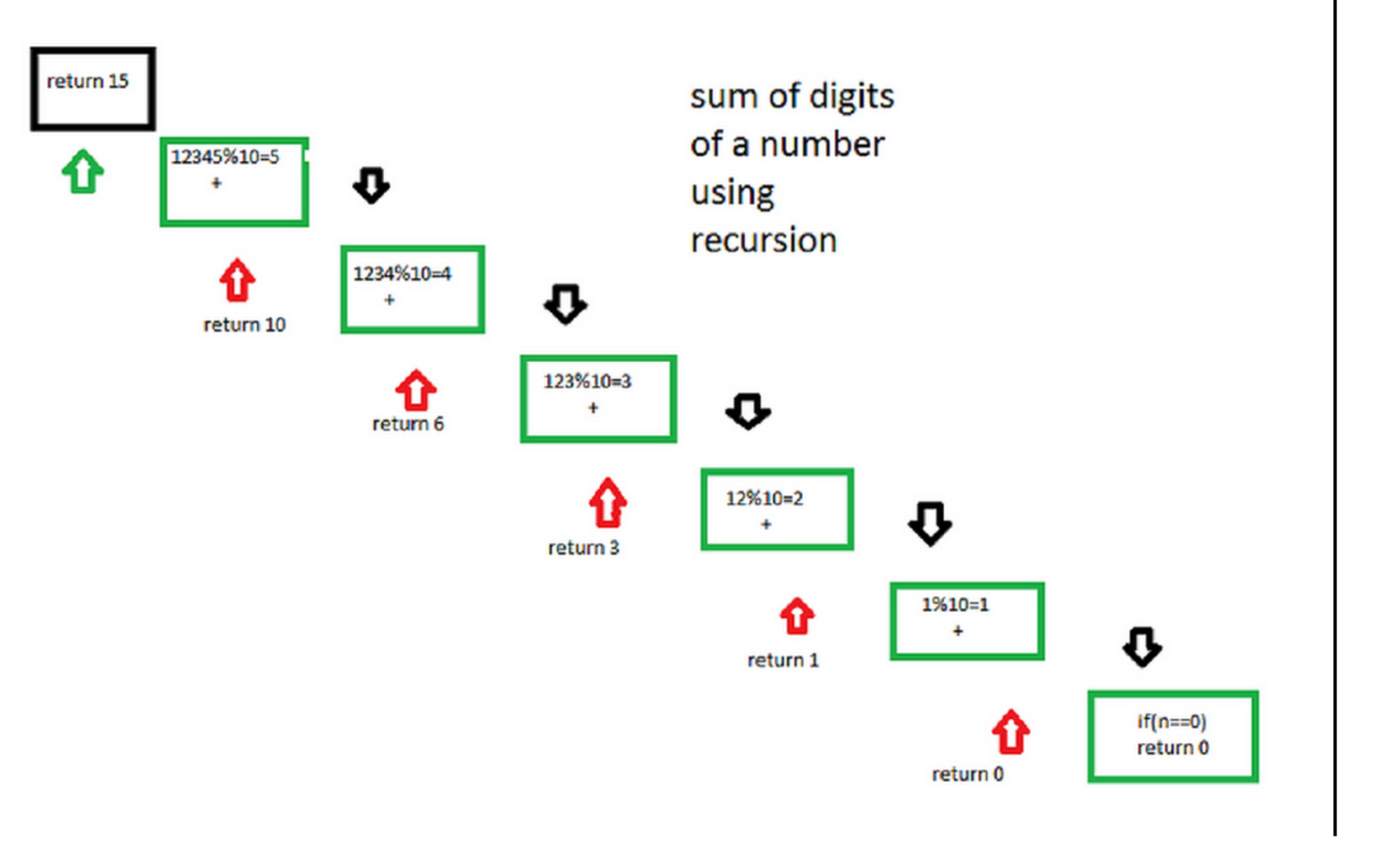# Sum of digit of a number using recursion

• Difficulty Level : Basic
• Last Updated : 16 Jun, 2022

Given a number, we need to find sum of its digits using recursion.
Examples:

```Input : 12345
Output : 15

Input : 45632
Output :20
```

The step-by-step process for a better understanding of how the algorithm works.
Let the number be 12345.
Step 1-> 12345 % 10 which is equal-too 5 + ( send 12345/10 to next step )
Step 2-> 1234 % 10 which is equal-too 4 + ( send 1234/10 to next step )
Step 3-> 123 % 10 which is equal-too 3 + ( send 123/10 to next step )
Step 4-> 12 % 10 which is equal-too 2 + ( send 12/10 to next step )
Step 5-> 1 % 10 which is equal-too 1 + ( send 1/10 to next step )
Step 6-> 0 algorithm stops
following diagram will illustrate the process of recursion## C++

 `// Recursive C++ program to find sum of digits``// of a number``#include ``using` `namespace` `std;` `// Function to check sum of digit using recursion``int` `sum_of_digit(``int` `n)``{``    ``if` `(n == 0)``    ``return` `0;``    ``return` `(n % 10 + sum_of_digit(n / 10));``}` `// Driven code``int` `main()``{``    ``int` `num = 12345;``    ``int` `result = sum_of_digit(num);``    ``cout << ``"Sum of digits in "``<< num``       ``<<``" is "``<

## C

 `// Recursive C program to find sum of digits``// of a number``#include ` `// Function to check sum of digit using recursion``int` `sum_of_digit(``int` `n)``{``    ``if` `(n == 0)``       ``return` `0;``    ``return` `(n % 10 + sum_of_digit(n / 10));``}` `// Driven Program to check above``int` `main()``{``    ``int` `num = 12345;``    ``int` `result = sum_of_digit(num);``    ``printf``(``"Sum of digits in %d is %d\n"``, num, result);``    ``return` `0;``}`

## Java

 `// Recursive java program to``// find sum of digits of a number``import` `java.io.*;` `class` `sum_of_digits``{``    ``// Function to check sum``    ``// of digit using recursion``    ``static` `int` `sum_of_digit(``int` `n)``    ``{``        ``if` `(n == ``0``)``            ``return` `0``;``        ``return` `(n % ``10` `+ sum_of_digit(n / ``10``));``    ``}` `    ``// Driven Program to check above``    ``public` `static` `void` `main(String args[])``    ``{``        ``int` `num = ``12345``;``        ``int` `result = sum_of_digit(num);``        ``System.out.println(``"Sum of digits in "` `+``                           ``num + ``" is "` `+ result);``    ``}``}` `// This code is contributed by Anshika Goyal.`

## Python3

 `# Recursive Python3 program to``# find sum of digits of a number` `# Function to check sum of``# digit using recursion``def` `sum_of_digit( n ):``    ``if` `n ``=``=` `0``:``        ``return` `0``    ``return` `(n ``%` `10` `+` `sum_of_digit(``int``(n ``/` `10``)))` `# Driven code to check above``num ``=` `12345``result ``=` `sum_of_digit(num)``print``(``"Sum of digits in"``,num,``"is"``, result)` `# This code is contributed by "Sharad_Bhardwaj".`

## C#

 `// Recursive C# program to``// find sum of digits of a number``using` `System;` `class` `GFG {``    ` `    ``// Function to check sum``    ``// of digit using recursion``    ``static` `int` `sum_of_digit(``int` `n)``    ``{``        ``if` `(n == 0)``            ``return` `0;``            ` `        ``return` `(n % 10 + sum_of_digit(n / 10));``    ``}` `    ``// Driven Program to check above``    ``public` `static` `void` `Main()``    ``{``        ``int` `num = 12345;``        ``int` `result = sum_of_digit(num);``        ``Console.WriteLine(``"Sum of digits in "` `+``                           ``num + ``" is "` `+ result);``    ``}``}` `// This code is contributed by Anant Agarwal.`

## PHP

 ``

## Javascript

 ``

Output:

`Sum of digits in 12345 is 15`

Besides writing (n==0 , then return 0) in the code given above we can also write it in this manner , there will be no change in the output .

`if(n<10) return n; By writing this there will be no need to call the function for the numbers which are less than 10 `

Time complexity : O(logn) where n is the given number.

Auxiliary space : O(logn) due to recursive stack space.

My Personal Notes arrow_drop_up